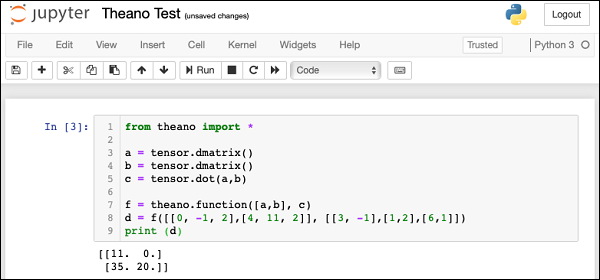# Theano - Expression for Matrix Multiplication

We will compute a dot product of two matrices. The first matrix is of dimension 2 x 3 and the second one is of dimension 3 x 2. The matrices that we used as input and their product are expressed here −

$$\begin{bmatrix}0 & -1 & 2\\4 & 11 & 2\end{bmatrix} \:\begin{bmatrix}3& -1 \\1 & 2 \\35 & 20 \end{bmatrix}=\begin{bmatrix}11 & 0 \\35 & 20 \end{bmatrix}$$

## Declaring Variables

To write a Theano expression for the above, we first declare two variables to represent our matrices as follows −

a = tensor.dmatrix()
b = tensor.dmatrix()


The dmatrix is the Type of matrices for doubles. Note that we do not specify the matrix size anywhere. Thus, these variables can represent matrices of any dimension.

## Defining Expression

To compute the dot product, we used the built-in function called dot as follows −

c = tensor.dot(a,b)


The output of multiplication is assigned to a matrix variable called c.

## Defining Theano Function

Next, we define a function as in the earlier example to evaluate the expression.

f = theano.function([a,b], c)


Note that the input to the function are two variables a and b which are of matrix type. The function output is assigned to variable c which would automatically be of matrix type.

## Invoking Theano Function

We now invoke the function using the following statement −

d = f([[0, -1, 2], [4, 11, 2]], [[3, -1],[1,2], [6,1]])


The two variables in the above statement are NumPy arrays. You may explicitly define NumPy arrays as shown here −

f(numpy.array([[0, -1, 2], [4, 11, 2]]),
numpy.array([[3, -1],[1,2], [6,1]]))


After d is computed we print its value −

print (d)


You will see the following output on the output −

[[11. 0.]
[25. 20.]]


## Full Program Listing

The complete program listing is given here:
from theano import *
a = tensor.dmatrix()
b = tensor.dmatrix()
c = tensor.dot(a,b)
f = theano.function([a,b], c)
d = f([[0, -1, 2],[4, 11, 2]], [[3, -1],[1,2],[6,1]])
print (d)


The screenshot of the program execution is shown here −